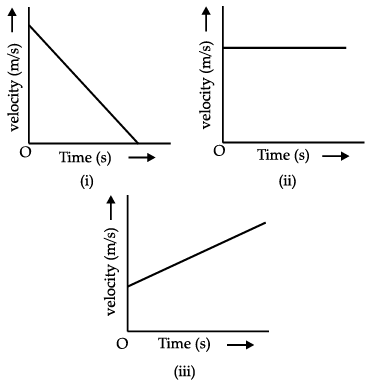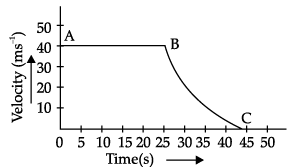Test: Motion- Case Based Type Questions- 1

# Test: Motion- Case Based Type Questions- 1 - Class 9

Test Description

## 10 Questions MCQ Test Science Class 9 - Test: Motion- Case Based Type Questions- 1

Test: Motion- Case Based Type Questions- 1 for Class 9 2023 is part of Science Class 9 preparation. The Test: Motion- Case Based Type Questions- 1 questions and answers have been prepared according to the Class 9 exam syllabus.The Test: Motion- Case Based Type Questions- 1 MCQs are made for Class 9 2023 Exam. Find important definitions, questions, notes, meanings, examples, exercises, MCQs and online tests for Test: Motion- Case Based Type Questions- 1 below.
Solutions of Test: Motion- Case Based Type Questions- 1 questions in English are available as part of our Science Class 9 for Class 9 & Test: Motion- Case Based Type Questions- 1 solutions in Hindi for Science Class 9 course. Download more important topics, notes, lectures and mock test series for Class 9 Exam by signing up for free. Attempt Test: Motion- Case Based Type Questions- 1 | 10 questions in 20 minutes | Mock test for Class 9 preparation | Free important questions MCQ to study Science Class 9 for Class 9 Exam | Download free PDF with solutions
 1 Crore+ students have signed up on EduRev. Have you?
Test: Motion- Case Based Type Questions- 1 - Question 1

### Direction: Identify the following graphs and answer any four questions :Q. The slope of the velocity-time graph gives the ___________.

Detailed Solution for Test: Motion- Case Based Type Questions- 1 - Question 1
The slope of the velocity-time graph gives the acceleration. n. When we plot velocity along the Y-axis and time along X- axis, the slope of the velocity time graph gives the acceleration produced.
Test: Motion- Case Based Type Questions- 1 - Question 2

### Direction: Identify the following graphs and answer any four questions :Q. What do you infer from the graph where velocity time graph is parallel to the time axis ?

Detailed Solution for Test: Motion- Case Based Type Questions- 1 - Question 2
When velocity time graph is parallel to time axis, velocity = constant, i.e., acceleration of body is zero.
Test: Motion- Case Based Type Questions- 1 - Question 3

### Direction: Identify the following graphs and answer any four questions :Q. Name the type of motion in which speed remains constant but the velocity of body changes.

Detailed Solution for Test: Motion- Case Based Type Questions- 1 - Question 3

The circular motion is the type of the motion of the body which has constant speed but not constant velocity because when a body moves in circular motion, the speed of the body remain constant all time of the motion but the direction changes continuously.

Test: Motion- Case Based Type Questions- 1 - Question 4

Direction: Identify the following graphs and answer any four questions :Q. Which of the graphs indicate negative acceleration? Why ?

Detailed Solution for Test: Motion- Case Based Type Questions- 1 - Question 4
If the object is slowing down then its acceleration vector is directed in the opposite direction as its motion (in this case, a negative acceleration). ... The acceleration-time graph shows a horizontal line in the negative region of the graph (meaning a negative acceleration).
Test: Motion- Case Based Type Questions- 1 - Question 5

Direction: Identify the following graphs and answer any four questions :Q. Which of the graphs represent a body moving with initial velocity not equal to zero but with constant acceleration?

Detailed Solution for Test: Motion- Case Based Type Questions- 1 - Question 5
Graph III; since the velocity-time graph does not pass through the origin.
Test: Motion- Case Based Type Questions- 1 - Question 6

Direction: Study the following graph and choose the correct options to answer any four questions given below:

The velocity-time graph of an object is shown in the following figure.Q. Which of the following statement is correct?

Detailed Solution for Test: Motion- Case Based Type Questions- 1 - Question 6
This is not possible because it would mean that velocity is increasing without increase in time i.e. acceleration is infinite and infinite acceleration is impossible.
Test: Motion- Case Based Type Questions- 1 - Question 7

Direction: Study the following graph and choose the correct options to answer any four questions given below:

The velocity-time graph of an object is shown in the following figure.Q. Identify the part of graph where the object has zero acceleration. Give reasons for your answer.

Detailed Solution for Test: Motion- Case Based Type Questions- 1 - Question 7
(a) As we know that if the slope of velocity time graph is parallel to time axis then the body is moving with a constant velocity.Hence, From A to B body has an constant velocity of 40 m/s

From B to C, the body uniformly retards as the velocity changes with respect to time.

(b) From A to B, the body has zero acceleration (i.e, retardation) as we know that if the body is uniform velocity acceleration automatically becomes zero here in the graph as we know the body started from 40 metre per second and from A to B, when body reaches at B its velocity remains 40 metre per second.Hence there is no change in velocity and we can say that acceleration is zero in this part of the graph

(c)From B to C, the body has negative acceleration (i.e, retardation) as we know that if the body has some initial velocity u and after sometime t if it gains some velocity v, we can say the body has an acceleration a.if the initial velocity is greater than the final velocity the acceleration becomes negative that is retardation of the body is seen.similarly here at point B if we considered the initial velocity to be 40 metre per second it uniformly retards to 0 m/s by the time it crosses 45 secs.

Test: Motion- Case Based Type Questions- 1 - Question 8

Direction: Study the following graph and choose the correct options to answer any four questions given below:

The velocity-time graph of an object is shown in the following figure.Q. State the kind of motion that objects has, from A to B and from B to C.

Detailed Solution for Test: Motion- Case Based Type Questions- 1 - Question 8
From A to B, the body has zero acceleration (i.e, retardation) as we know that if the body is uniform velocity acceleration automatically becomes zero here in the graph as we know the body started from 40 metre per second and from A to B, when body reaches at B its velocity remains 40 metre per second.Hence there is no change in velocity and we can say that acceleration is zero in this part of the graph

From B to C, the body has negative acceleration (i.e, retardation) as we know that if the body has some initial velocity u and after sometime t if it gains some velocity v, we can say the body has an acceleration a.if the initial velocity is greater than the final velocity the acceleration becomes negative that is retardation of the body is seen.similarly here at point B if we considered the initial velocity to be 40 metre per second it uniformly retards to 0 m/s by the time it crosses 45 secs.

Test: Motion- Case Based Type Questions- 1 - Question 9

Direction: Study the following graph and choose the correct options to answer any four questions given below:

The velocity-time graph of an object is shown in the following figure.Q. Identify the part of graph where the object has negative acceleration. Give reasons for your answer.

Detailed Solution for Test: Motion- Case Based Type Questions- 1 - Question 9
From A to B, the body has zero acceleration (i.e, retardation) as we know that if the body is uniform velocity acceleration automatically becomes zero here in the graph as we know the body started from 40 metre per second and from A to B, when body reaches at B its velocity remains 40 metre per second.Hence there is no change in velocity and we can say that acceleration is zero in this part of the graph

(c) From B to C, the body has negative acceleration (i.e, retardation) as we know that if the body has some initial velocity u and after sometime t if it gains some velocity v, we can say the body has an acceleration a.if the initial velocity is greater than the final velocity the acceleration becomes negative that is retardation of the body is seen.similarly here at point B if we considered the initial velocity to be 40 metre per second it uniformly retards to 0 m/s by the time it crosses 45 secs.

Test: Motion- Case Based Type Questions- 1 - Question 10

Direction: Study the following graph and choose the correct options to answer any four questions given below:

The velocity-time graph of an object is shown in the following figure.Q. The area enclosed by the velocity-time graph and the time axis represents the __________.

Detailed Solution for Test: Motion- Case Based Type Questions- 1 - Question 10
The area enclosed by the velocity-time graph and the time axis represents the displacement.

Area enclosed by a velocity-time sketch with time axis represents velocity × time = displacement.

Velocity being a vector quantity, area under time axis corresponds to negative velocity × time = negative displacement.

## Science Class 9

66 videos|352 docs|97 tests
Information about Test: Motion- Case Based Type Questions- 1 Page
In this test you can find the Exam questions for Test: Motion- Case Based Type Questions- 1 solved & explained in the simplest way possible. Besides giving Questions and answers for Test: Motion- Case Based Type Questions- 1, EduRev gives you an ample number of Online tests for practice

## Science Class 9

66 videos|352 docs|97 tests